Next: The Intermediate Vector Boson Up: Examples Previous: The Hadronic Current

## Unitarity Limit

For the phenomenological treatment of weak interactions we have the current-current effective Lagrangian (77). It is found that the known semileptonic and leptonic processes are well described by the matrix elements of the Lagrangian at first order of perturbation theory. Nevertheless, there are doubts about the behaviour of higher orders. That is to say, the renormalization properties (of theoretical interest) of the theory so formulated. It is found that the theory is not renormalizable because too many divergences appear. So it is valid to question where, at which energy scale, these divergences will turn unsustainable. That is to say where this model will stop having any value, even a phenomenological one.

A typical process to study is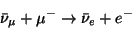(101)

whose first order cross section, as a dimensional analysis shows, is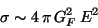(102)

On the other hand, the optical theorem at high energies allows to write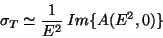(103)

where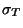is the total cross section for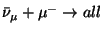and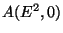is the amplitude for elastic forward dispersion which is written, without taking into account the spin dependence, from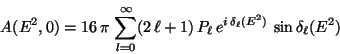(104)

But in the process we are dealing with, one finds that in the angular distribution only the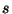waves are important, therefore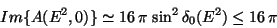(105)

Taking this result to (105) we have: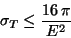(106)

and so expression (104), calculated perturbatively at first order, can be correct only for energies such that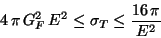(107)

that is to say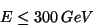(108)

called unitarity limit as it is provided by the optical theorem. In conclusion, from a certain energy of the order given by (110), the limit imposed by unitarity is violated and the effective model of four fermions point interaction lacks sense.

In order to obtain some inspiration to continue it is interesting to compare the behaviour of a similar process but this time of the quantum electrodynamics. For instance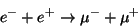(109)

In this case the interaction is electromagnetic and is mediated by the photon: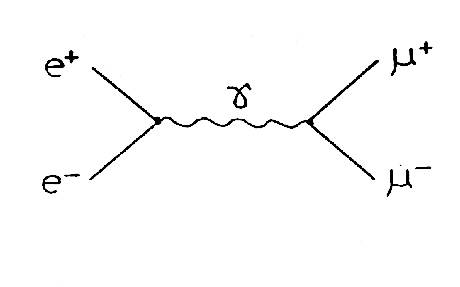and the interaction terms are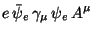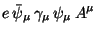The first order cross section of the process results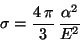(110)

decreasing with the energy as unitarity requires. The conclusions of this comparison will be clearer from a short dimensional analysis. Under the election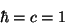(111)

it results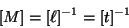(112)

and the exponent of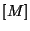is called dimension. Then it results, for example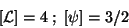(113)

In particular one has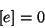(114)

which turns evident the origin of the behaviour (112).

Summarizing, inspiration came: the necessity of searching for a theory of weak interactions involving a dimensionless coupling constant and a mediator of the interaction is clear.Next: The Intermediate Vector Boson Up: Examples Previous: The Hadronic Current
root 2001-01-22